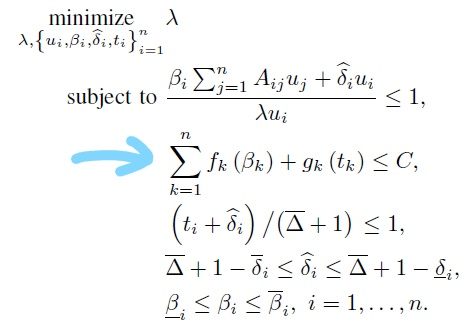Illegal operation: {convex} + {concave}Here in the marked constraint : “fk(beta_k)” is convex and “gk(t_k)” is concave function .But while I am using it in CVX matlab code facing error :

Disciplined convex programming error:
Illegal operation: {convex} + {concave}

How to solve this error

In order for that constraint to be convex, the LHS needs to be convex. As with your previous question, we are not mindreaders, so we don’t know what f_k and g_k are; therefore we can’t be sure that the LHS is not convex. But until you show us otherwise, I will presume so.

I have provided the following link to you before. You need to read it carefully, as your current post does not illustrate that you have understood it.

Maybe you should study at least the first 5 or so chapters of https://web.stanford.edu/~boyd/cvxbook/bv_cvxbook.pdf .in order to learn some basics about convex optimization.

here fk is convex function and gk is concave

Why does that fact imply you model is convex?

For instance

f(x) =x^2
g(x)=-2x^2

does not imply

f(x)+g(x)

is convex.

I have not told my model is convex. I am asking how can we find sum of concave+convex. Is it possible or not

You can model that in CVX only if the LHS is convex. For instance, if f(x) = 2x^2, g(x) = -x^2, then f(x) + g(x) = x^2, which is convex, and can be entered in CVX as x^2, but it can’t be entered in CVX as 2x^2-x^2.

Again, I implore you to read the link, which obviously you have not understood.

1 Like